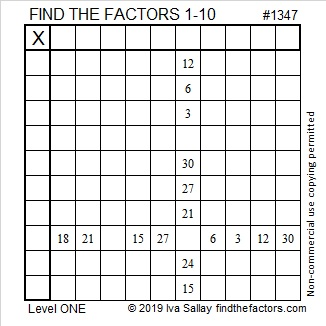# 1347 and Level 1

Which ten division facts do you need to know to solve this puzzle? Seriously, you can do this one!Print the puzzles or type the solution in this excel file: 10 Factors 1347-1356

If you’re not sure how to solve it, I explain how in this youtube video:

Here are some facts about the number 1347:

• 1347 is a composite number.
• Prime factorization: 1347 = 3 × 449
• 1347 has no exponents greater than 1 in its prime factorization, so √1347 cannot be simplified.
• The exponents in the prime factorization are 1, and 1. Adding one to each exponent and multiplying we get (1 + 1)(1 + 1) = 2 × 2 = 4. Therefore 1347 has exactly 4 factors.
• The factors of 1347 are outlined with their factor pair partners in the graphic below.1347 isn’t a Lucas number, but as OEIS.org reminds us, 1, 3, 4, 7 happens to be the first four Lucas numbers. Here’s why: